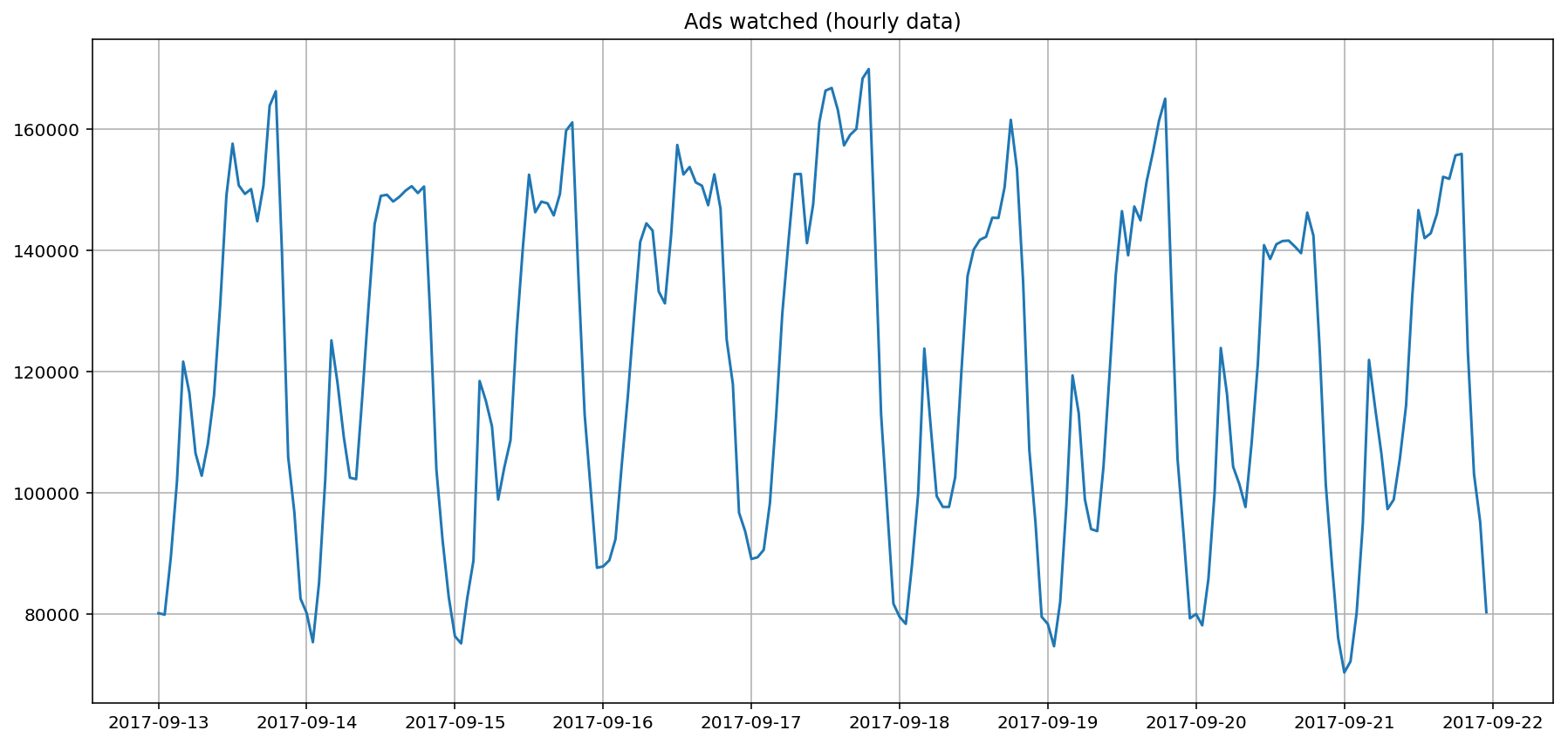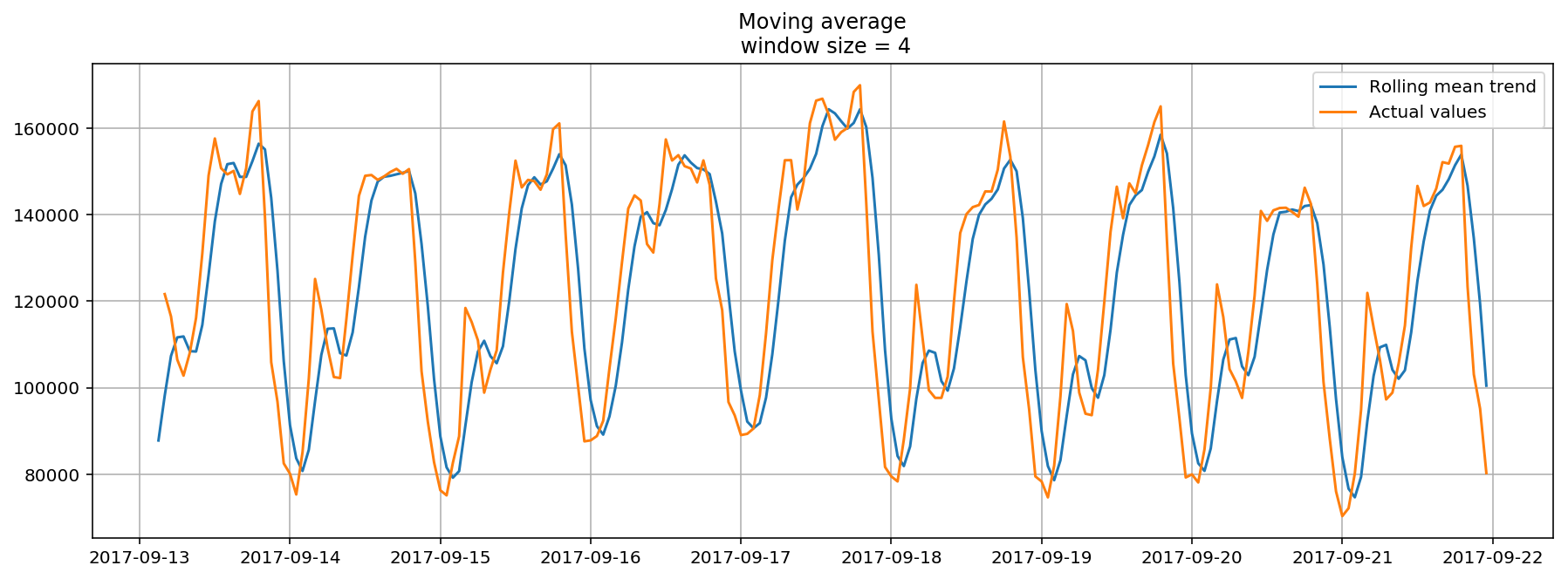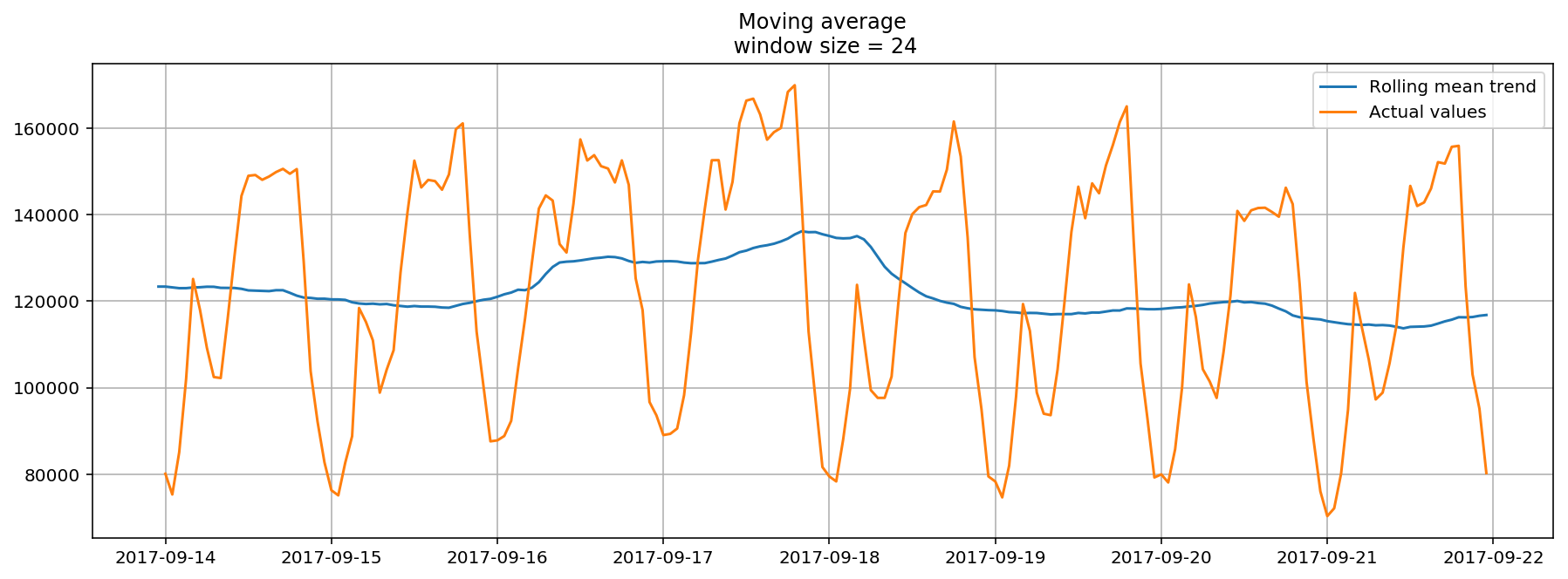In :
# code for loading the format for the notebook
import os

# path : store the current path to convert back to it later
path = os.getcwd()
os.chdir(os.path.join('..', 'notebook_format'))


Out:
In :
os.chdir(path)

# 1. magic for inline plot
# 2. magic to print version
# 3. magic so that the notebook will reload external python modules
# 4. magic to enable retina (high resolution) plots
# https://gist.github.com/minrk/3301035
%matplotlib inline
%config InlineBackend.figure_format='retina'

import numpy as np
import pandas as pd
import matplotlib.pyplot as plt
from scipy.optimize import minimize
from sklearn.metrics import mean_squared_log_error
from sklearn.model_selection import TimeSeriesSplit

%watermark -a 'Ethen' -d -t -v -p numpy,scipy,pandas,sklearn,matplotlib

Ethen 2018-08-22 12:37:16

CPython 3.6.4
IPython 6.4.0

numpy 1.14.1
scipy 1.1.0
pandas 0.23.0
sklearn 0.19.1
matplotlib 2.2.2


# Getting Started with Time Series Analysis¶

The gist behind time series analysis is that we are given some quantitative measures about the past and we wish to use these informations to predict the future to enable better planning, decision-making and so on. The main difference between time series problem and traditional prediction problems is that: in traditional prediction problems such as image classification, the data points there are assumed to be independent of one another. Whereas, time series analysis' data points have a temporal nature in them, i.e. The time dimension adds an explicit ordering to our data points that should be preserved because they can provide additional/important information to the learning algorithms.

As an example, we will look at a real mobile game data that depicts ads watched per hour.

In :
ads_col = 'Ads'
time_col = 'Time'

dimension:  (216, 1)

Out:
Time
2017-09-13 00:00:00 80115
2017-09-13 01:00:00 79885
2017-09-13 02:00:00 89325
2017-09-13 03:00:00 101930
2017-09-13 04:00:00 121630
In :
plt.figure(figsize=(15, 7))
plt.grid(True)
plt.show()## Averages¶

To make a prediction of the next point given this time series, one of the most naive method that we can use is the arithmetic average of all the previously observed data points. We take all the values we know, calculate the average and bet that that's going to be the next value. Of course it won't be it exactly, but it probably will be somewhere in the ballpark (e.g. your final school grade may be the average of all the previous grades).

\begin{align} \hat{y}_{x+1} = \dfrac{1}{x}\sum_{i=1}^{x}y_i \end{align}
• Where $\hat{y}_{x+1}$ refers to the predicted value at time $x + 1$
In :
def average(series):
return np.mean(series)

average(series)

Out:
121974.05092592593

An improvement over simple arithmetic average is the average of $n$ last points. The rationale here is that only recent values matter and adding previous data points into consideration would only be adding noise. Calculation of the moving average involves what is sometimes called a "sliding window" of size $n$.

\begin{align} \hat{y}_{x+1} = \dfrac{1}{x} \sum_{i=x - n}^{x}y_i \end{align}
In :
def moving_average(series, n):
"""Calculate average of last n observations"""
return np.mean(series[-n:])

# prediction for the last observed day (past 24 hours)
moving_average(series, 24)

Out:
116805.0

Although we can't really use this method for making predictions really far out into the future (because in order to get the value for the next step, we need the previous values to be actually observed), the moving average method can be used to smooth the original time series for spotting trend. As we'll soon see, the wider the window, the smoother the trend.

In :
def plot_moving_avg(series, window):
rolling_mean = series.rolling(window=window).mean()

plt.figure(figsize=(15,5))
plt.title('Moving average\n window size = {}'.format(window))
plt.plot(rolling_mean, label='Rolling mean trend')

plt.plot(series[window:], label='Actual values')
plt.legend(loc='best')
plt.grid(True)While it might not seem that useful when we set the window size to be 4, if we were to apply the smoothing on a 24 hour window, we would get a daily trend that shows us a more interesting and perhaps expected pattern. That is: during the weekends, the values are higher (more time to play on the weekends?) while fewer ads are watched on weekdays.

In :
plot_moving_avg(ads, window=24)Side note, the following code chunk shows an implementation of moving average without using pandas' rolling functionality.

In :
def moving_average(arr, window):
"""
http://stackoverflow.com/questions/14313510/how-to-calculate-moving-average-using-numpy
"""
ret = np.cumsum(arr)
ret[window:] = ret[window:] - ret[:-window]
return ret[window - 1:] / window

arr = np.arange(20)
print(moving_average(arr, window=4))

[ 1.5  2.5  3.5  4.5  5.5  6.5  7.5  8.5  9.5 10.5 11.5 12.5 13.5 14.5
15.5 16.5 17.5]


## Exponential Smoothing¶

Now let's see what happens if, instead of only weighting the time series' last $k$ values, we would weight all available observations while exponentially decreasing the weights as we move further back in time.

A weighted moving average is a moving average where within the sliding window values are given different weights, typically so that more recent points matter more. Instead of only weighting the time series' last $k$ values, however, we could instead consider all of the data points, while assigning exponentially smaller weights as we go back in time. This method is so called Exponential Smoothing. The mathematical notation for this method is:

\begin{align} \hat{y}_x = \alpha \cdot y_x + (1 - \alpha) \cdot \hat{y}_{x-1} \end{align}

To compute the formula, we pick an $0 < \alpha < 1$ and a starting value $\hat{y}_{0}$ (i.e. the first value of the observed data), and then calculate $\hat{y}_x$ recursively for $x = 1, 2, 3, \dots$. As we'll see in later sections, $\hat{y}_x$ is also referred to as levels.

We can think of $\alpha$ as the smoothing factor or memory decay rate, it defines how quickly we will "forget" the last available true observation. The smaller $\alpha$ is, the more influence the previous observations have and the smoother the series is. In other words, the higher the $\alpha$, the faster the method "forgets" about the past.

In :
def exponential_smoothing(series, alpha):
"""given a series and alpha, return series of expoentially smoothed points"""
results = np.zeros_like(series)

# first value remains the same as series,
# as there is no history to learn from
results = series
for t in range(1, series.shape):
results[t] = alpha * series[t] + (1 - alpha) * results[t - 1]

return results

In :
def plot_exponential_smoothing(series, alphas):
"""Plots exponential smoothing with different alphas."""
plt.figure(figsize=(15, 7))
for alpha in alphas:
plt.plot(exponential_smoothing(series, alpha), label='Alpha {}'.format(alpha))

plt.plot(series, label='Actual')
plt.legend(loc='best')
plt.axis('tight')
plt.title('Exponential Smoothing')
plt.grid(True)

plot_exponential_smoothing(series.values, [0.3, 0.05])